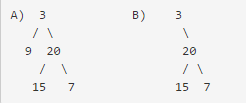# Balanced Binary Tree Problem

Problem description:
level: medium

Given a binary tree, determine if it is height-balanced.

For this problem, a height-balanced binary tree is defined as a binary tree in which the depth of the two subtrees of every node never differ by more than 1.

Example
Given binary tree A={3,9,20,#,#,15,7}, B={3,#,20,15,7}The binary tree A is a height-balanced binary tree, but B is not.

Solution:

consider a node, if left is balanced, right is balanced, and left, right nodes height difference < 2, then the node is balanced

Another thought:
if a node is not balanced, then its height set to -1
else return it is real height

Using Divide & Conquer, we can get the height to decide if it is a balanced tree

Time complex: O(n)